# GSEB Solutions Class 6 Maths Chapter 11 Algebra Ex 11.1

Gujarat Board GSEB Textbook Solutions Class 6 Maths Chapter 11 Algebra Ex 11.1 Textbook Questions and Answers.

## Gujarat Board Textbook Solutions Class 6 Maths Chapter 11 Algebra Ex 11.1

Question 1.
Find the rule which gives the number of matchsticks required to make the following matchsticks patterns. Use a variable to write the rule.
(a) A pattern of letter T as(b) A pattern of letter Z as(c) A pattern of letter U as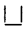(d) A pattern of letter V as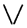(e) A pattern of letter E as(f) A pattern of letter S as(g) A pattern of letter A as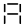Solution:
(a)A number of matchsticks required:
For n = 1 is 2 or 2 × n
For n = 2 is 4 or 2 × n
For n = 3 is 6 or 2 × n
For n = 4 is 8 or 2 × n
∴ Rule: 2n

(b)A number of matchsticks:
For n = 1 is 3 or 3 × n
For n = 2 is 6 or 3 × n
For n = 3 is 9 or 3 × n
For n = 4 is 8 or 3 × n
∴ Rule: 3n

(c)A number of matchsticks:
For n = 1 is 3 or 3 × n
For n = 2 is 6 or 3 × n
For n = 3 is 9 or 3 × n
For n = 4 is 12 or 3 × n
∴ Rule: 3n

(d)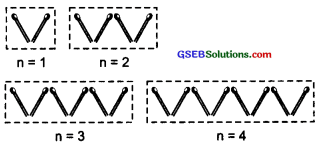A number of matchsticks:
For n = 1 is 2 or 2 × n
For n = 2 is 4 or 2 × n
For n = 3 is 6 or 2 × n
For n = 4 is 8 or 2 × n
∴ Rule: 2n

(e)A number of matchsticks:
For n = 1 is 5 or 5 × n
For n = 2 is 10 or 5 × n
For n = 3 is 15 or 5 × n
∴ Rule: 5n

(f)A number of matchsticks:
For n = 1 is 5 or 5 × n
For n = 2 is 10 or 5 × n
For n = 3 is 15 or 5 × n
∴ Rule: 5n

(g)A number of matchsticks:
For n = 1 is 6 or 6 × n
For n = 2 is 12 or 6 × n (∴ Rule : 6n)
For n = 3 is 18 or 6 × n
∴ Rule: 6nQuestion 2.
We already know the rule for the pattern of letters L, C, and F. Some of the letters from Q.1 (given above) give us the same rule as that given by L. Which are these? Why does this happen?
Solution:
The rules to find the number of matchsticks for:
The letter L is 2n The letter C is 3n
The letter V is 2n The letter U is 3 n
The letter T is 2 n The letter F is 4n
It is the same for L, V, and T. The number of matchsticks required in each of them is 2.

Question 3.
Cadets are marching in a parade. There are 5 cadets in a row. What is the rule which gives the number of cadets, given the number of rows? (Use n for the number of rows.)
Solution:
Number of rows = n
Number of cadets in a row = 5n = 1, is 5 or 5 × n
n = 2, is 10 or 5 × n
n = 3, is 15 or 5 × n
The rule to find the cadets in n rows is 5n.

Question 4.
If there are 50 mangoes in a box, how will you write the total number of mangoes in terms of the number of boxes? (Use b for the number of boxes.)
Solution:
Number of mangoes in a box = 50
∴ Number of mangoes, when
b = 1, is 50 or 50 × 1 = 50 × b
[‘b’ stands for a box]
b = 2, is 100 or 50 × 2 = 50 × b
b = 3, is 150 or 50 × 3 = 50 × b
Thus, total number of mangoes are 50b.Question 5.
The teacher distributes 5pencils per student. Can you tell me how many pencils are needed, given the number of students? (Use s for the number of students.)
Solution:
A number of students are represented by ‘s’.
Number of pencils distributed per student = 5
Now, number of pencils, when
s = 1, is 5 or 5 × 1 = 5 × s
s = 2, is 10 or 5 × 2 = 5 × s
s = 3, is 15 or 5 × 3 = 5 × s
∴ Number of pencils for s students is 5s.Question 6.
A bird flies 1 kilometer in one minute. Can you express the distance covered by the bird in terms of its flying time in minutes? (Use t for flying time in minutes.)
Solution:
∵ The flying time is represented by ‘t’.
Distance covered in one minute = 1 km
∴ Distance covered, when
t = 1, is 1 km or 1 × 1 km = 1 × t km
t = 2, is 2 km or 1 × 2 km = 1 × t km
t = 3, is 3 km or 1 × 3 km = 1 × t km
Thus, distance covered in ‘t’ minutes = t km.Question 7.
Radha is drawing a dot Rangoli (a beautiful pattern of lines joining dots with chalk powder). She has 9 dots in a row. How many dots will her Rangoli have for r rows? How many dots are there if there are 8 rows? If there are 10 rows?
Solution:
A number of rows are given by ‘r’.
∵ The number of dots in a row is 9.
∴ A total number of dots:
For r = 1 is 9   or 9 × 1 = 9 × r
For r = 2 is 18 or 9 × 2 = 9 × r
For r = 3 is 27 or 9 × 3 = 9 × r
Thus, the total number of dots is given by 9r.
Now, For r = 8, we have 9 × 8 = 72 dots
For r = 10, we have 9 × 10 = 90 dots.Question 8.
Leela is Radha s younger sister. Leela is 4 years younger than Radha. Can you write Leela s age in terms of Radha s age? Take Radha’s age to be x years.
Solution:
Let the age of Radha = x years
Since the age of Leela = (Age od Radha) – 4 years
Leela’s age = x years – 4 years = (x – 4) years.

Question 9.
Solution:
Number of laddus leftover = 5
Number of laddus given away = l
∴ Total number of laddus made by mother = l + 5Question 10.
Oranges are to be transferred from larger boxes into smaller boxes. When a large box is emptied, the oranges from it fill two smaller boxes and still, 10 oranges remain outside. If the number of oranges in a small box is taken to be x, what is the number of oranges in the larger box?
Solution:
Number of oranges in a large box = 2x
[Number of oranges in a small box] + 10
[∵ 10 oranges remain outside]
Since, x represents the number of oranges in the smaller box
∴ Number of oranges in a large box = 2[x] + 10 = 2x + 10

Question 11.
(a) Look at the following matchstick pattern of squares (Fig. I). The squares are not separate. Two neighboring squares have a common matchstick. Observe the patterns and find the rule that gives the number of matchsticks in terms of the number of squares. (Hint: If you remove the vertical stick at the end, you will get a pattern of Cs.)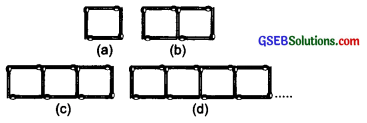(b) Fig. II gives a matchstick pattern of triangles. As in Q. 11(a) above, find the general rule that gives the number of matchsticks in terms of the number of triangles.Solution:
Let n be the number of squares or triangles
(a) ∴ Number of matchsticks, when
n = 1 is 4   or 3 × 1 + 1 = 3n + 1
n = 2 is 7   or 3 × 2 + 1 = 3n + 1
n = 3 is 10  or 3 × 3 + 1 = 3n + 1
n = 4 is 13  or 3 × 4 + 1 = 3n + 1
Thus, the required rule is 3n + 1.
(b) Number of matchsticks, when
n = 1 is 3   or 2 × 1 + 1 = 2 n + 1
n = 2 is 5   or 2 × 2 + 1 = 2 n + 1
n = 3 is 7   or 2 × 3 + 1 = 2 n + 1
n = 4 is 9   or 2 × 4 + 1 = 2 n + 1
Thus, the required rule is 2n + 1.